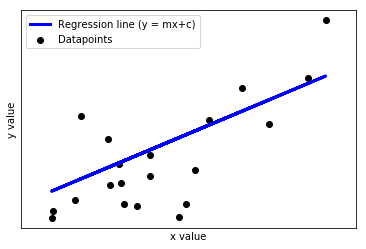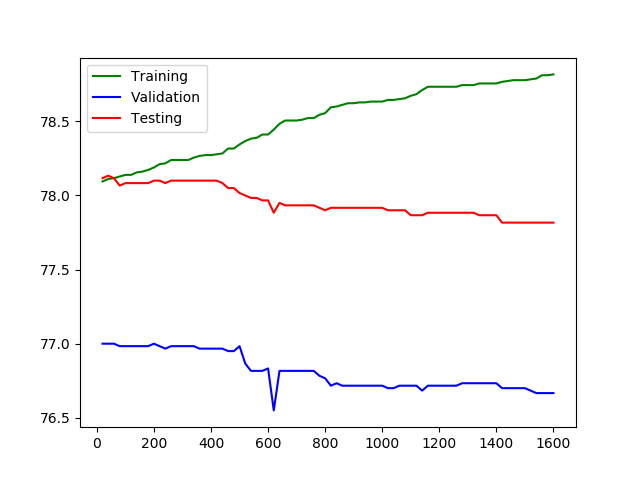Skip to content
Related Articles
Difference Between Model Parameters VS HyperParameters
• Last Updated : 17 May, 2020

The two most confusing terms in Machine Learning are Model Parameters and Hyperparameters. In this post, we will try to understand what these terms mean and how they are different from each other.

## What is a Model Parameter?

A model parameter is a variable of the selected model which can be estimated by fitting the given data to the model.

Example:In the above plot, x is the independent variable, and y is the dependent variable. The objective is to fit a regression line to the data. This line(the model) is then used to predict the y-value for unseen values of x. Here, m is the slope and c is the intercept of the line. These two parameters(m and c) are estimated by fitting a straight line to the data by minimizing the RMSE(root mean squared error). Hence, these parameters are called the model parameters.

Model parameters in different models:

• m(slope) and c(intercept) in Linear Regression
• weights and biases in Neural Networks

## What is a Model Hyperparameter?

A model hyperparameter is the parameter whose value is set before the model start training. They cannot be learned by fitting the model to the data.

Example:In the above plot x-axis represents the number of epochs and y-axis represents the number of epochs. We can see after the certain point when epochs are more than then although the traitning accuracy increases but the validation and test accuracy starts decreasing. This is a case of overfishing. Here number of epochs is a hyperparameter and is is set manually. Setting this number to a small value may cause underfitting and high value may cause overfitting.

Model hyperparameters in different models:

• Learning rate in gradient descent
• Number of iterations in gradient descent
• Number of layers in a Neural Network
• Number of neurons per layer in a Neural Network
• Number of clusters(k) in k means clustering

## Table of difference between Model Parameters and HyperParameters

PARAMETERSHYPERPARAMETER
They are required for making predictionsThey are required for estimating the model parameters
They are estimated by optimization algorithms(Gradient Descent, Adam, Adagrad)They are estimated by hyperparameter tuning
They are not set manuallyThey are set manually
The final parameters found after training will decide how the model will perform on unseen dataThe choice of hyperparameters decide how efficient the training is. In gradient descent the learning rate decide how efficient and accurate the optimization process is in estimating the parametersMy Personal Notes arrow_drop_up